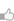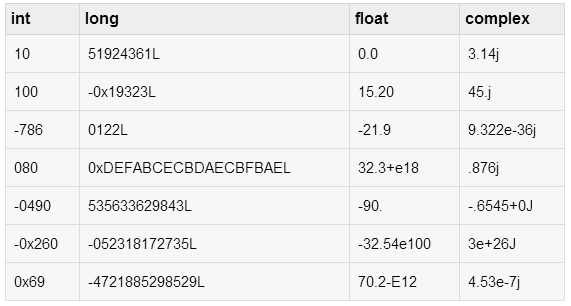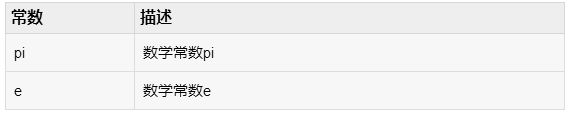求知 文章 文库 Lib 视频 iProcess 课程 认证 咨询 工具 讲座 Modeler Code

 Python教程 Python快速入门 Python概述 Python环境安装 Python基本语法 Python变量类型 Python基本运算符 Python决策 Python循环 Python数据类型 Python字符串 Python列表 Python元组 Python字典 Python日期时间Date/Time Python函数 Python模块 Python文件I/O Python异常处理 高级教程 Python 3开发网络爬虫(一) Python 3开发网络爬虫(二) Python 3(三): 伪装浏览器 Python 3(四): 登录 Python面向对象 Python正则表达式 Python CGI编程
Python数字类型
 355 次浏览24次捐助

 `var1 = 1var2 = 10`

 `del var1[,var2[,var3[....,varN]]]]`

 `del vardel var_a, var_b`

Python支持四种不同的数值类型：

int (有符号整数): 通常被称为只是整数或整数，是正或负整数，不带小数点。

long (长整数 ): 或长，是无限大的整数，这样写整数，后面跟着一个大写或小写的L。

float (浮点实数值) : 或浮点数，表示实数，并写入一个小数点分隔的整数部分和小数部分。浮点数也可以是科学记数法，用e或E表示的功率10 (2.5e2 = 2.5 x 102 = 250).

complex (复数) : 形式如 a + bJ，其中a和b是浮点和J（或j）表示-1的平方根（这是一个虚数）。 a是数的实部，b是虚部。Python编程不使用复杂的数字。Python允许长整型使用一个小写L，但建议只使用一个大写的L避免与数字1看起来一样，Python显示长整数用一个大写L。

Python中含有混合类型计算表达式内部将数字转换为普通类型。但有时，需要从一种类型的显式强制数到另一个类型，以满足操作符或函数参数的要求。

Python包括以下执行数学计算的函数。

 函数 返回（描述） abs(x) x的绝对值：x和零之间的（正极）的距离。 ceil(x) x的上限：最小整数不小于x cmp(x, y) -1 if x < y, 0 if x == y, 或1 if x > y exp(x) x的指数: ex fabs(x) x的绝对值 floor(x) x的地板：最大的整数不大于x log(x) x的自然对数，对于x> 0时 log10(x) 以10为底的对数，X>0。 max(x1, x2,...) 它最大的参数：值最接近正无穷大 min(x1, x2,...) 它的最小参数：值最接近负无穷大 modf(x) x的两个项元组的整数和小数部分。这两个元素具有相同的x符号。整数部分返回一个浮点数 pow(x, y) x**y 的值 round(x [,n]) x在小数点四舍五入到n位数字。 Python远离零点决定：round(0.5) 是1.0 而round(0.5) 为-1.0 sqrt(x) x的平方根（x>0）

 函数 描述 choice(seq) 从列表，元组或字符串随机项。 randrange ([start,] stop [,step]) 从范围随机选择的元素（启动，停止，步骤）。 random() 随机浮点数r，使得0是小于或等于r，r小于1 seed([x]) 设置生成随机数使用整数开始值。调用任何其他随机模块函数之前调用这个函数。返回None。 shuffle(lst) 随机化代替列表中的项。返回None。 uniform(x, y) 随机浮点数r，使得x小于或等于r，r小于y

Python包括以下执行三角函数计算功能。

 函数 描述 acos(x) 返回x的反余弦值，以弧度形式表示 asin(x) 返回x的反正弦，以弧度形式表示 atan(x) 返回x的反正切值，以弧度表示形式 atan2(y, x) 返回反正切atan(y / x)，以弧度形式表示 cos(x) 返回x 弧度的余弦 hypot(x, y) 返回欧几里德范数，sqrt(x*x + y*y) sin(x) 返回x的弧度的正弦值 tan(x) 返回x的弧度的正切 degrees(x) 从弧度到度角 x 的转换 radians(x) 从角度到弧度角 x 的转换您可以捐助，支持我们的公益事业。 1元 10元 50元 认证码：必填
 355 次浏览24次捐助